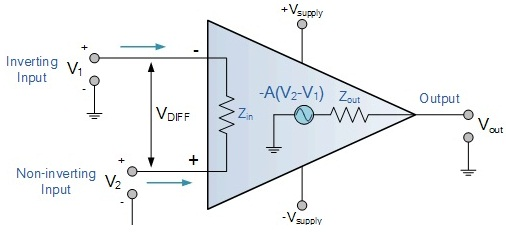Logarithmic Amplifier

Objective

To design and study a Logarithmic Amplifier using Op-amp 741

Introduction

Log amplifier is a linear circuit in which the output voltage will be a constant times the natural logarithm of the input. The basic output equation of a log amplifier is v Vout = K ln ( Vin / Vref ); where Vref is the constant of normalization and K is the scale factor. Log amplifier finds a lot of application in electronic fields like multiplication or division (they can be performed by the addition and subtraction of the logs of the operand), signal processing, computerized process control, compression, decompression, RMS value detection etc.

The term "Logarithmic Amplifier" (generally abbreviated to "log amp") is something of a misnomer, and "Logarithmic Converter" would be a better description. The conversion of a signal to its equivalent logarithmic value involves a nonlinear operation, the consequences of which can be confusing if not fully understood. It is important to realize that many of the familiar concepts of linear circuits are irrelevant to log amps. For example, the incremental gain of an ideal log amp approaches infinity as the input tends to zero, and a change of offset at the output of a log amp is equivalent to a change of amplitude at its input—not a change of input offset.

Basically there are two log Op-amp configurations: Op-amp diode log amplifier and Op-amp transistor log.

Ideal Op-amp: The figure below shows an example of an ideal operational amplifier. The main part in an amplifier is the dependent voltage source that increases in relation to the voltage drop across Rin, thus amplifying the voltage difference between V + and V -. Many uses have been found for Op-amp and an ideal Op-amp seeks to characterize the physical phenomena that make Op-amps useful.Fig.1 Op-amp 741Fig.2 Ideal Op-amp

Vs+ and Vs- are not connected to the circuit within the Op-amp because they power the dependent voltage source’s circuit. These are notable, however, because they determine the maximum voltage the dependent voltage source can output. For any input voltage the ideal Op-amp has,
a. Infinite open-loop gain
b. Infinite bandwidth
c. Infinite input impedance
d. Zero offset voltage
e. Infinite slew rate
f. Zero output impedance and
g. Zero noise